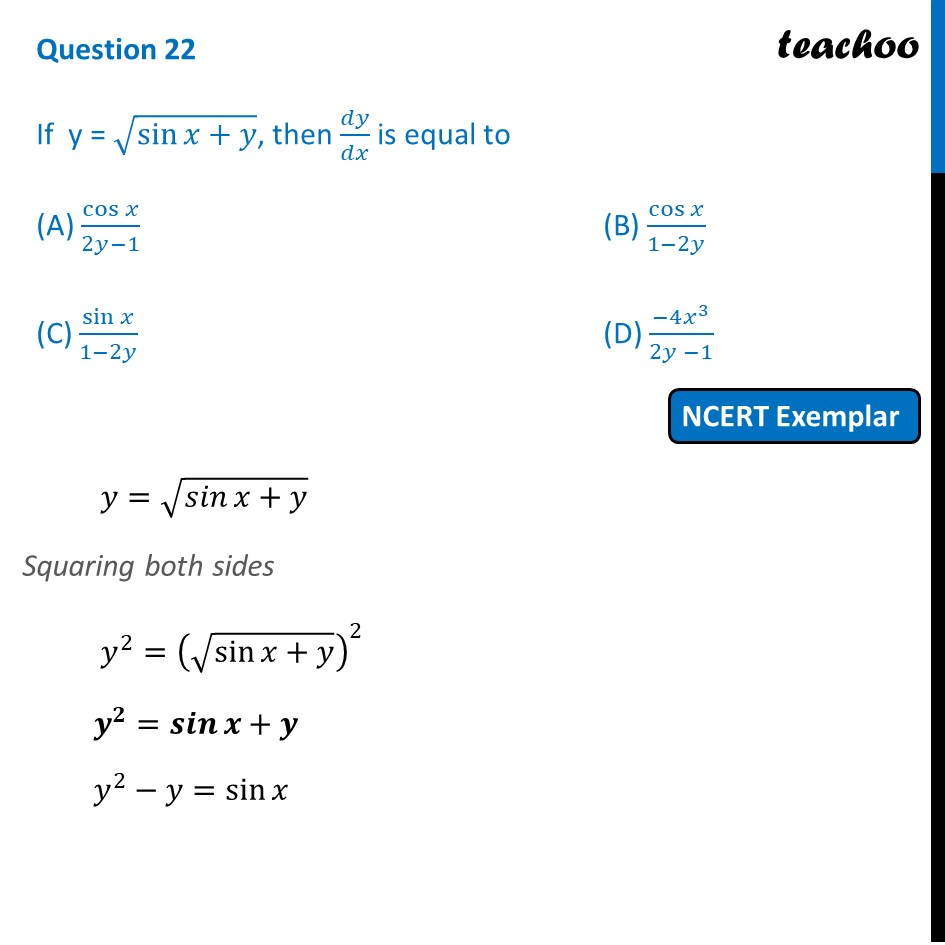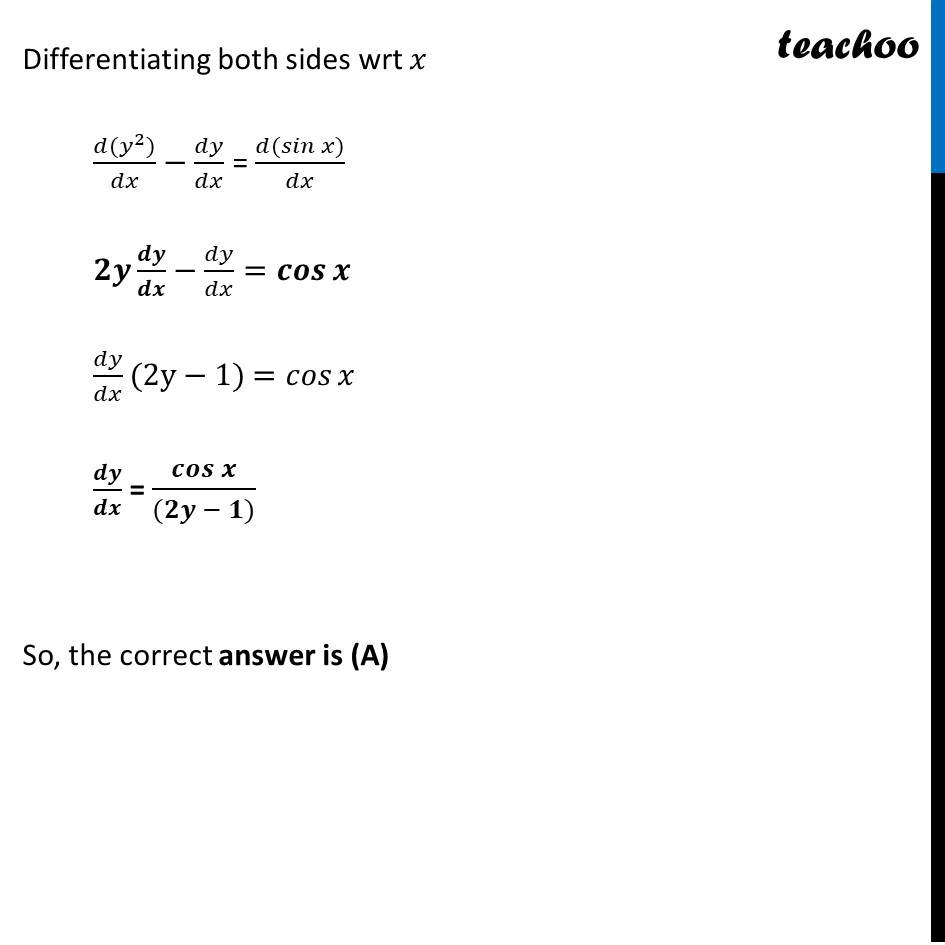NCERT Exemplar - MCQs

Chapter 5 Class 12 Continuity and Differentiability
Serial order wise

## (D) (-4x 3 )/(2y -1)

This question is similar to Example 25 - Chapter 5 Class 12 - Continuity and DifferentiabilityLearn in your speed, with individual attention - Teachoo Maths 1-on-1 Class

### Transcript

Question 20 If y = √(sin⁡〖𝑥+𝑦〗 ), then 𝑑𝑦/𝑑𝑥 is equal to (A) cos⁡𝑥/(2𝑦−1) (B) cos⁡𝑥/(1−2𝑦) (C) sin⁡𝑥/(1−2𝑦) (D) (−4𝑥^3)/(2𝑦 −1) 𝑦=√(𝑠𝑖𝑛⁡〖𝑥+𝑦〗 ) Squaring both sides 𝑦^2=(√(sin⁡〖𝑥+𝑦〗 ))^2 𝒚^𝟐=𝒔𝒊𝒏⁡〖𝒙+𝒚〗 𝑦^2−𝑦=sin⁡𝑥 Differentiating both sides wrt 𝑥 (𝑑〖(𝑦〗^2))/𝑑𝑥−𝑑𝑦/𝑑𝑥 = (𝑑(𝑠𝑖𝑛⁡〖𝑥)〗)/𝑑𝑥 𝟐𝒚 𝒅𝒚/𝒅𝒙−𝑑𝑦/𝑑𝑥=𝒄𝒐𝒔⁡𝒙 𝑑𝑦/𝑑𝑥(2y−1) =𝑐𝑜𝑠⁡𝑥 𝒅𝒚/𝒅𝒙 = 𝒄𝒐𝒔⁡𝒙/((𝟐𝒚 − 𝟏)) So, the correct answer is (A)